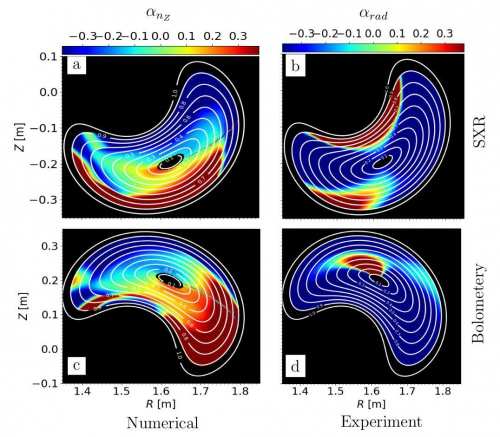# TJ-II:Impurity density and potential asymmetries

## Experimental campaign

2017 SpringFigure 1. Numerical vs Experiment electron-root #45477 discharge: (a,b) Numerical impurity density asymmetry parameter $\alpha_{nZ}$ and the experimental radiation asymmetry parameter $\alpha_{rad}$ respectively at the toroidal plane $\phi=14.5^{\circ}$ that corresponds the SXR toroidal measurement plane. (c,d) Numerical impurity density asymmetry parameter $\alpha_{nZ}$ and the experimental radiation asymmetry parameter $\alpha_{rad}$ respectively at the toroidal plane $\phi=75.5^{\circ}$ that corresponds the Bolometery toroidal position. Note the numerical and experimental scale sare the same $[-0.38, 0.38]$. Since neon impurities were puffed at trave levels during the discharges, the numerical results in (a,c) have considered $Z_{I}=10$, i.e. they have assumed fully ionization of Ne on the whole effective radius 

## Proposal title

Impurity density and potential asymmetries

## Name and affiliation of proponent

José M García Regaña1, M. Ezzat1, B. van Milligen1, M. A. Ochando 1, F. Medina1, José Luis Velasco1, J. A. Alonso 1, I. Calvo 1, C. Hidalgo1, K. McKarthy1

1. Fusion National Laboratory, CIEMAT, 28040, Madrid, Spain

## Description of the activity, including motivation/objectives and experience of the proponent (typically one-two pages)

Neoclassical theory predicts a non-constant portion of the electrostatic potential over the flux surfaces , usually denoted by $\Phi_1=\Phi_1(\theta,\phi)$, with $\theta$ and $\phi$ the poloidal and toroidal angular coordinates. When this is taken into account the equilibrium density of the different species a present in the plasma varies according to their adiabatic response and can be written as: $n_{a0}=\left<n\right>\exp\left(-Z_{a}e\Phi_1/T_{a}\right)$, with $\left<...\right>$ the flux-surface-average. In TJ-II plasmas experiments and simulations    have shown that $e\Phi_1/T_{a}$ can take values from $O(0.01)$ to $O(0.1)$. Variations are predicted to be larger at the outer radii than at the inner core, and stronger in ECRH plasmas than in NBI plasmas. Under conditions with large $\Phi_1$ the impurities of moderate to high $Z$ should experience strong variations of their densities over the flux surfaces, increasing with $Z$. These, in turn, should result in an anisotropic radiation over each flux surface and consequently a radially asymmetric radiation pattern should follow.

In the present experiment the analysis of the radial profiles and time evolution of the plasma emissivity using the TJ-II bolometry system  after the inyection of some selected impurities by gas puffing is proposed. The experiment aims at studying the above-mentioned link between the radially assymetric emissivity and the measured and predicted $\Phi_1$. The measurement and evolution of $\Phi_1$ will be tracked during the discharges using the duplicated Langmuir probe system plasma floating potential measurements. Numerical calculations of $\Phi_1$ will be carried out with the neoclassical version of the code EUTERPE at different radial locations. The application of fluid tools is also foreseen for the comparison between simulations and with the experimental results.

## Description of required resources

The required signals to perform the analysis are:

• The time evolution of the plasma emissivity radial profile via tomographic reconstructions of the bolometry system signals.
• The time evolution of the plasma floating potential at the outer core region ($r/a\sim 0.9$).
• The time evolution of the line-averaged density $\left<n_e(t)\right>$ with interferometry.
• The radial profiles of electron density $n_{e}(r, t_0)$ and temperature at one time instant $t_0$ using Thomson Scattering (TS).
• The time evolution of the electron temperature profile $T_{e}(r,t)$ with Electron Cyclotron Emission (ECE), when available, calibrated with TS.
• The time evolution of the ion temperature in the core and in an outer radial position $T_i(r/a\sim 0.2,t)$ and $T_i(r/a\sim 0.8,t)$ with the Neutral Particle Analyzer (NPA).
• The time evolution of the radial electric field at the mid-outer resion ($r/a\sim 0.7-0.8$) with reflectometry.
• The time evolution of the electrostatic potential in the mid-outer region $\Phi(r/a\sim0.7,t)$ with the double Heavy Ion Beam Probe (HIBP).

Other constraints regarding the desired experimental conditions are:

• Good reproducibility of the plasma discharges to allow comparison across impurity and charge states $Z$. Scannig $T_e$ is also considered by the application of different ECH injected power.
• Good stationarity of plasma parameters at the instant where the impurities are injected is required in order to extract the stationary background emissivity from that produced by the injected impurity. Hence the study shall preferably be perform in ECRH plasmas.

## Preferred dates and degree of flexibility

Preferred dates: (format dd-mm-yyyy)